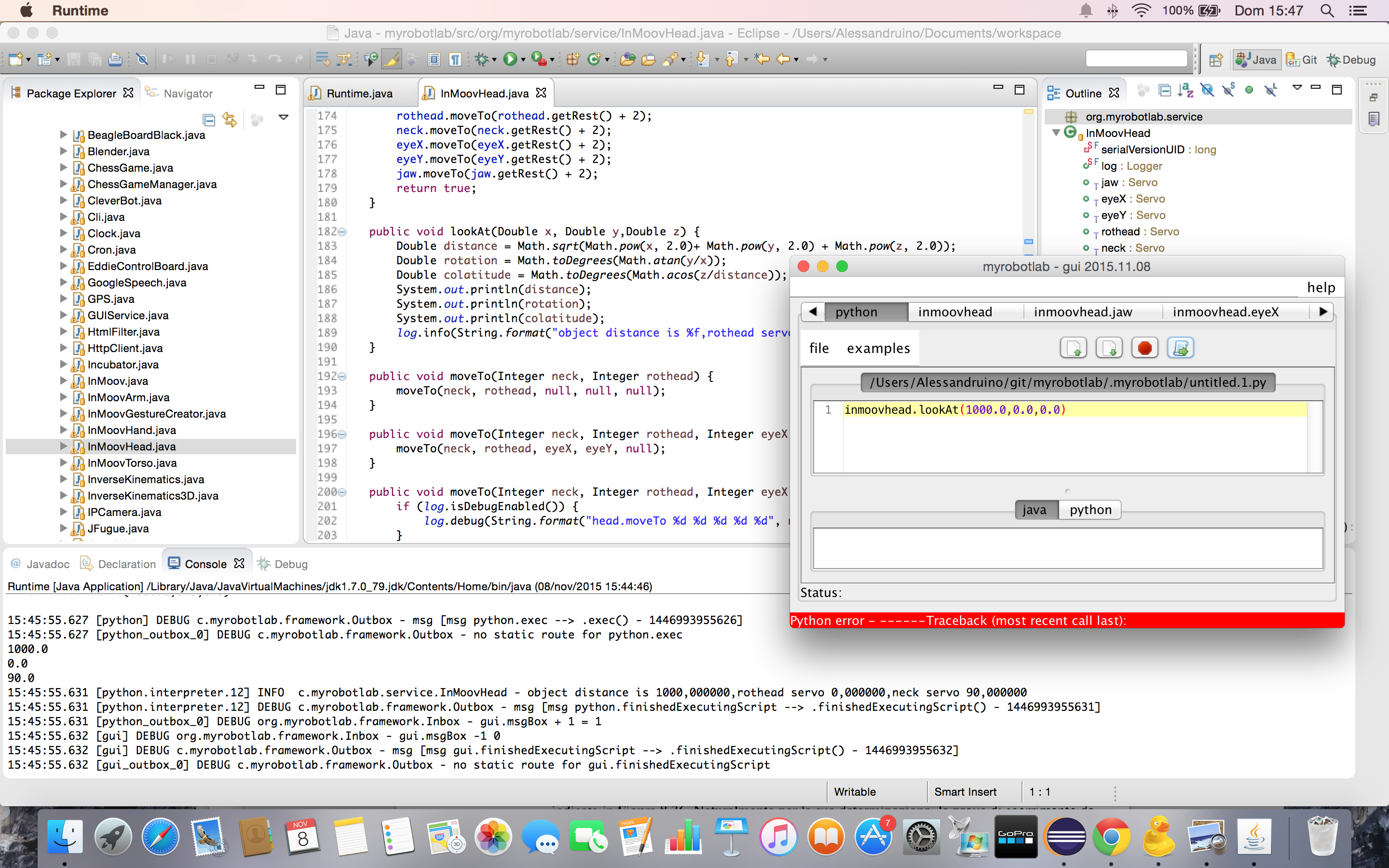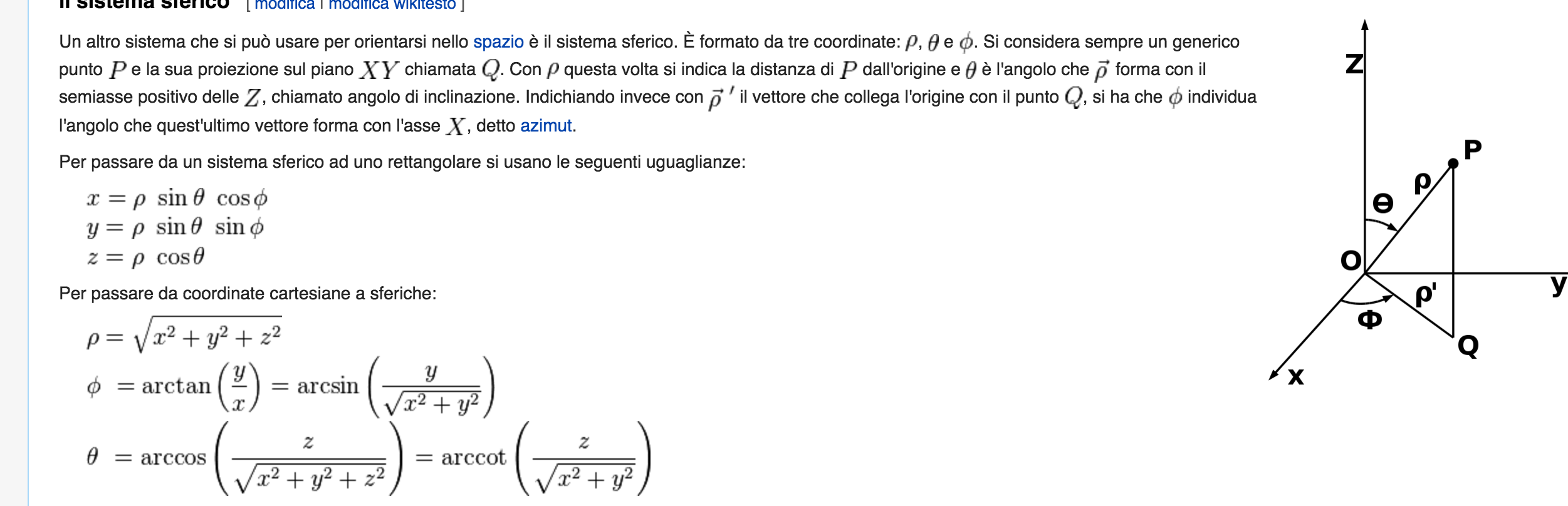# eyes following hand

Given that MRL knows all my movement commands and servo angles - it should theoretically be possible to set the eyes/head to follow or point to the left or right hand position? Or is this already available?### Hey juerg... you want to

Hey juerg... you want to "fake it" or you want accurate pointing?

What i mean is , if you want accurate pointing then we need to do some geometric calculs about direct kinematics, and head orientation...

if you want to fake it, just map servos of the head to move accrording to arm movement.

Ale### Hi Ale, hope you had a good

Hi Ale, hope you had a good time in Rome, at least it looks like that in the video.

Now - the fake/accurate question was not really a question???

I assume It would add up nicely to have some built in "intelligence" in InMoov as  I found having to script all the servo angles might be fun for a while but tends to get tedious.

So the challenge as you noted would be to do the proper kinetics calculatioins and follow the hand wherever it goes?

I have seen once a video of InMoov playing bowling so the basic kinetics should be available? Might be a huge task so...

Juerg### Hey juerg... I added this to

public void lookAt(Double x, Double y,Double z) {

Double distance = Math.sqrt(Math.pow(x, 2.0)+ Math.pow(y, 2.0) + Math.pow(z, 2.0));

Double rotation = Math.toDegrees(Math.atan(y/x));

Double colatitude = Math.toDegrees(Math.acos(z/distance));

System.out.println(distance);

System.out.println(rotation);

System.out.println(colatitude);

log.info(String.format("object distance is %f,rothead servo %f,neck servo %f ", distance, rotation, colatitude));}

This is one step closer to what you were asking for...

This method translate the (x,y,z) coordinate of the hand of the robot or of an object in the 3d Space , and translate it in a spherical system of coordinated with the center of inmoov head ( distance of the object, rothead  and neck)Now we just need the InverseKinematics Service by Kevin to provide coordinates of the palm of the hand and send it to this little code in inmoovhead :) and we are done :)